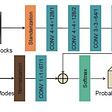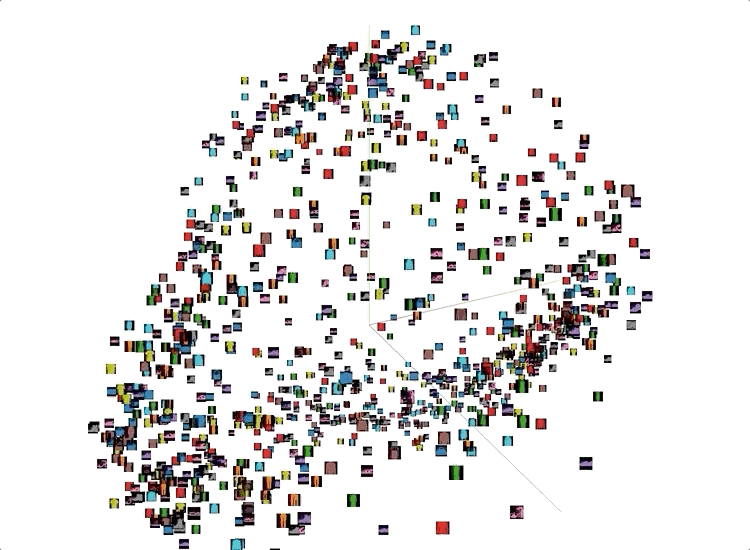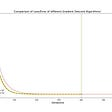# Random Forest ML Algorithm

There are many machine learning algorithms used for supervised machine learning. One popular machine learning algorithm is the Random Forest algorithm.

Random Forest (RF) is an ensemble machine learning algorithm. Ensemble machine learning algorithms utilize the advantage of using the prediction of multiple algorithms. Ensemble techniques include boosting and bagging techniques.

Random Forest is a bagging ensemble machine learning algorithm.

In bagging, different machine learning models can be used. However, in Random Forest, there are only multiple decision trees present. RF can be used in both regression and classification tasks.

## Random Forest Classifier

The main Decision Tree is that it is subject to overfitting which leads to low bias and high variance. With RF, we aim to avoid this and achieve a low bias and a low variance.
In an RF Classifier, the data is both row and column sampled and given to each decision tree. An important thing to note here is that there can be an overlap of data received by each decision tree. This is also termed bootstrap aggregation, which is used to reduce the variance with a noisy dataset. Finally, each decision tree is trained on the sampled train data. During the test phase, the output of each decision tree is collected and majority voting is applied to achieve the output class.

## Random Forest Regressor

As mentioned above the Random Forest is capable of regression also. A similar approach as that of the Random Forest Classifier is used in this case as well. The difference lies in the output stage. In this case of the Random Forest Regressor, the mean of the outputs of all the decision trees is taken.

## Random Forest Coding Sample

Let us look into a sample code for solving the IRIS classification problem using Random Forest. For loading and performing Exploratory Data Analysis (EDA) on the IRIS dataset, kindly refer to my earlier blog here.

Let’s fetch the data:

`from sklearn.datasets import load_irisimport pandas as pddata = load_iris() X = pd.DataFrame(data.data, columns=data.feature_names)y = pd.DataFrame(data.target, columns=["target"])df = pd.concat([X, y], axis=1)print(df.head())`

`# Perform Train-Test Splitfrom sklearn.model_selection import train_test_splitX_train, X_test, y_train, y_test = train_test_split(X, y, test_size=0.25, random_state=42)`

Let us create an object of Random Forest Classifier from sklearn. More information of the parameters can be found here.

`from sklearn.ensemble import RandomForestClassifiermodel = RandomForestClassifier(max_depth=5, n_estimators=10)`

It is very important to understand the internal parameters of the random forest algorithm as they play a huge role in avoiding overfitting. We have not optimized the algorithm in this case, as this is for demo purposes. Let us now, fit the data on this random forest model, and test it on the test data.

`from sklearn.metrics import classification_reportmodel.fit(X_train, y_train)y_pred = model.predict(X_test)print(classification_report(y_test, y_pred))`

It is evident from the classification matrix that the random forest classifier is doing a great job of classifying the IRIS species.

Add on Information: It is not required to perform normalization in a Random Forest Algorithm.

--

--

--

## More from Analytics Vidhya

Analytics Vidhya is a community of Analytics and Data Science professionals. We are building the next-gen data science ecosystem https://www.analyticsvidhya.com

## Top 3 Telegram groups for Machine learning that no one is talking about## Reading: CNNIF & CNNMC — Image Compression Using VVC, 1st & 2nd Places in CVPR 2018 CLIC (Codec…## Face Recognition Using VGG16## Milvus in Action: Building a Reverse Image Search System Based on Milvus and VGG## Applied Machine Learning: Part 1## Machine Learning Workflow for Research Scientists## Intro to Visual RecSys## Alternative distributional semantics approachFull-Stack Data Scientist

## Simple Linear Regression from Scratch!## Heatmap For Correlation Matrix & Confusion Matrix | Extra Tips On Machine Learning## Regression Regularization Techniques — Ridge and Lasso## SMS spam classification using Naïve Bayes Classifier